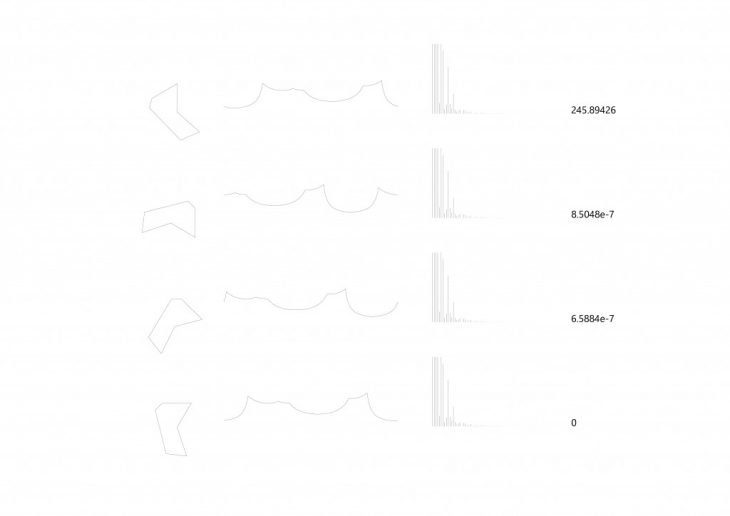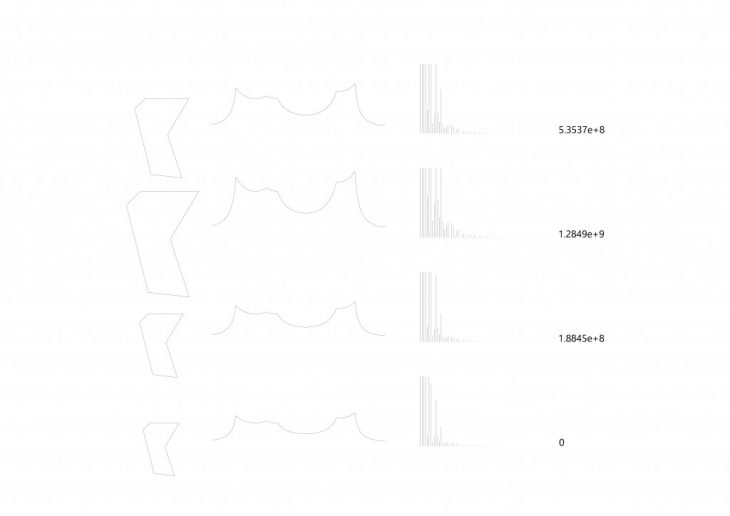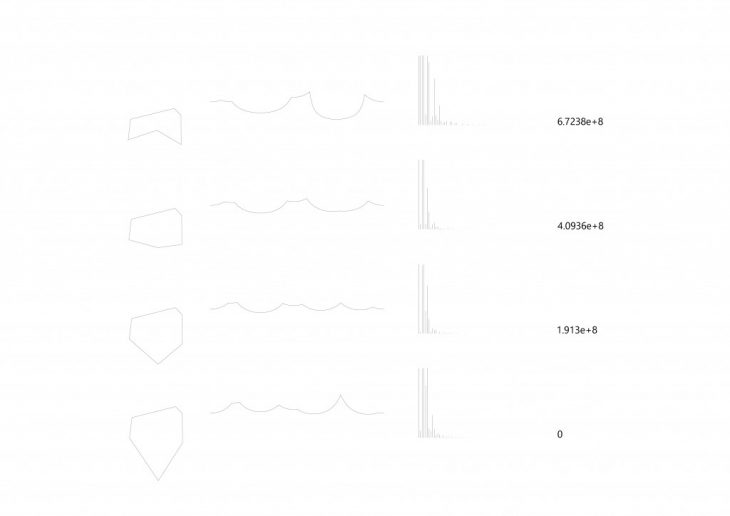This project is part of a former project in IAAC. The goal is to find a numerical representation of polygon shapes which enables the comparison of different polygons in terms of similarity through an automated process.
This numerical representation should be able to represent the shapes using a fixed length vector not matter what is the number of vertices or edges. In the context of this project, polygonal shapes are meant to be grouped based on their similarities in terms of size and shape. This requires the numerical representation to represent the shape “Rotation-Invariant” and “Scale-Dependant”.

For this reason, a polar representation of the shape is taken to the Cartesian system. This converts the closed shape into a repetitive signal. This signal can then be analyzed using Fourier Transform for its frequencies and the FT result can represent the polygon free of its rotation, as the rotation of the shape results in a shift in the signal which does not affect the result of the FT analysis.

Here are the results of some test polygons:
The polygons in each image are compared to the bottom-most polygon.